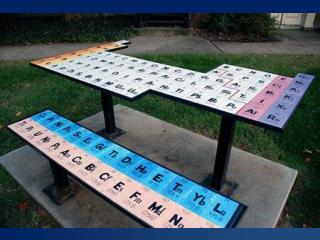# Teaching Stoichiometry in 60 Minutes or Less - PowerPoint PPT PresentationDownload PresentationTeaching Stoichiometry in 60 Minutes or Less

Teaching Stoichiometry in 60 Minutes or LessDownload Presentation## Teaching Stoichiometry in 60 Minutes or Less

- - - - - - - - - - - - - - - - - - - - - - - - - - - E N D - - - - - - - - - - - - - - - - - - - - - - - - - - -
##### Presentation Transcript

1. Teaching Stoichiometry in 60 Minutes or Less Stacey Cool CSTA, San Jose Oct 2012 Golden Valley High School Merced, CA scool@muhsd.org @CoolChemGal www.tinyurl.com/coolschem

2. Objective • Demonstrate a way to teach molar conversions, stoichiometry & other conversions using ratios

3. Background • Teach Chemistry & AP Chemistry for 10 years • In Merced, CA • Stoichiometry stumbling blocks CSTA, San Jose 2012; S. Cool

4. Why? • Issues with dimensional analysis • Power Standard • CSTA 2 years ago

5. Power Standard

6. The Process • What are you looking for, what are you given? • What you are looking for on top • What you are given on the bottom • Carry the units over • Fill in numbers • Cross multiply • Solve

7. November Molar Conversions

8. 22.4 L Volume (L) The Mole Road Map 22.4 L Mole Molar mass (periodic table) 6.02 x 1023 Mass (grams) Particles (atoms, molecules, formula units)

9. Some practice problems: • How many moles is 5.69 g of NaOH?

10. Some practice problems: • How many moles is 5.69 g of NaOH? x mol NaOH 1 mol NaOH = 5.69 g NaOH 40.00 g NaOH We need a relationship between grams & moles. Need to figure out the molar mass of NaOH! NaOH = 22.99 g/mol + 16.00 g/mol + 1.01 g/mol Molar mass of NaOH = 40.00 g/mol 1 mole NaOH= 40.00 g NaOH Cross multiply and solve for x. (x mol NaOH) = (5.69 g NaOH)(1 mol NaOH) 40.00 g NaOH = 0.14225 moles NaOH 3 sig figs = 0.142 moles NaOH

11. Some practice problems: • Determine the number of moles in 128.14 g of SO2.

12. Some practice problems: • Determine the number of moles in 128.14 g of SO2. x mol SO2 1 mol SO2 = 128.14 g SO2 64.07 g SO2 We need a relationship between grams & moles. Need to figure out the molar mass of SO2! SO2 = 32.07 g/mol + 2(16.00 g/mol) Molar mass of SO2 = 64.07 g/mol 1 mole SO2 = 64.07 g SO2 Cross multiply and solve for x. (x mol SO2) = (128.14 g SO2)(1 mol SO2) 64.07 g SO2 = 2 moles SO2 4 sig figs = 2.000 moles SO2

13. Examples What would 3.0 moles of carbon weigh?

14. January Stoichiometry

15. 22.4 L Volume (L) The Mole Road Map 22.4 L Mole Molar mass (periodic table) 6.02 x 1023 Mass (grams) Particles (atoms, molecules, formula units)

16. Mass (grams) Mass (grams) Molar mass (periodic table) Molar mass (periodic table) mole ratio Volume (L) Volume (L) Mole Mole 22.4 L 22.4 L Particles (atoms, molecules, formula units) Particles (atoms, molecules, formula units) 6.02 x 1023 6.02 x 1023

17. An Example Na + Cl2 2NaCl How many grams of sodium chloride are produced from 46 g of sodium and excess chlorine?

18. An Example Na + Cl2 2NaCl 2 molx x gNaCl g NaCl 58.5 = 1 molx g Na 46 g Na 23 (x g NaCl)= (46 g Na)(2 mol)(58.5 gNaCl) (1 mol) (23 g Na) = 117 g NaCl

19. An example... If 56 g of Fe are added to a solution of Copper (II) Sulfate, how much solid copper (MW = 64) will form? 2Fe + 3CuSO4Fe2(SO4)3 + 3Cu

20. Mass to Mole Zn + Cu2SO4 ZnSO4 + 2Cu How many moles of copper can be produced from 446 g of Cu2SO4(molar mass of Cu2SO4= 223 g/mol)?

21. Volume to Volume C6H12 + 9 O2 6CO2 + 6H2O How many liters of oxygen are needed to produce 20.0L of carbon dioxide?

22. Mole to Mole How many moles of O2 are produced when 3.00 moles of Al2O3 decompose? 2 Al2O3® 4Al+3O2

23. Practice 2 Al(s) + 6 HCl(aq)2 AlCl3(aq) + 3 H2(g) • When 23.0g of solid aluminum is reacted with excess hydrochloric acid, what volume of hydrogen gas is produced at STP?

24. Practice 2Al(s) + 6HCl(aq) 2AlCl3(aq) + 3H2(g) • Using the same 23.0g of aluminum as above, how many moles of HCl need to be added in order for it to react completely?

25. Practice 2Al(s) + 6HCl(aq) 2AlCl3(aq) + 3H2(g) • How many moles of aluminum chloride are produced from 0.60 mol of hydrochloric acid?

26. Other Applications • Pressure conversions • Joule-calorie conversions • Metric conversions Remember: You are teaching a problem solving process!

27. Converting Between Pressures • 1 atm = 760 mm Hg = 760 torr = 101.3 kPa • Convert 1520 torrto atm

28. Contact Info scool@muhsd.org @CoolChemGal www.tinyurl.com/coolschem CSTA, San Jose 2012; S. Cool